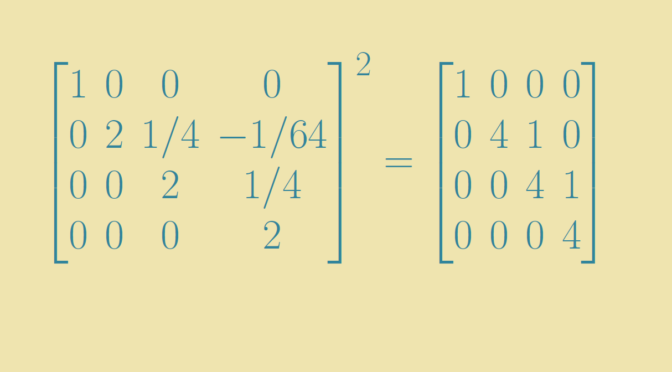Complex matrix without a square root

Consider for $$n \ge 2$$ the linear space $$\mathcal M_n(\mathbb C)$$ of complex matrices of dimension $$n \times n$$. Is a matrix $$T \in \mathcal M_n(\mathbb C)$$ always having a square root $$S \in \mathcal M_n(\mathbb C)$$, i.e. a matrix such that $$S^2=T$$? is the question we deal with.

First, one can note that if $$T$$ is similar to $$V$$ with $$T = P^{-1} V P$$ and $$V$$ has a square root $$U$$ then $$T$$ also has a square root as $$V=U^2$$ implies $$T=\left(P^{-1} U P\right)^2$$.

Diagonalizable matrices

Suppose that $$T$$ is similar to a diagonal matrix $D=\begin{bmatrix} d_1 & 0 & \dots & 0 \\ 0 & d_2 & \dots & 0 \\ \vdots & \vdots & \ddots & 0 \\ 0 & 0 & \dots & d_n \end{bmatrix}$ Any complex number has two square roots, except $$0$$ which has only one. Therefore, each $$d_i$$ has at least one square root $$d_i^\prime$$ and the matrix $D^\prime=\begin{bmatrix} d_1^\prime & 0 & \dots & 0 \\ 0 & d_2^\prime & \dots & 0 \\ \vdots & \vdots & \ddots & 0 \\ 0 & 0 & \dots & d_n^\prime \end{bmatrix}$ is a square root of $$D$$.

Invertible matrices

A complex matrix $$T$$ is similar to a matrix $J=\begin{bmatrix} J_1 & & \\ & \ddots &\\ & & J_p \end{bmatrix}$ where each block $$J_i$$ is a square matrix of the form $J_i=\begin{bmatrix} \lambda_i & 1 & &\\ & \lambda_i & \ddots &\\ & & \ddots & 1\\ & & & \lambda_i \end{bmatrix}$ $$J$$ is called the Jordan normal form of $$T$$. If $$T$$ is supposed to be invertible, the $$\lambda_i$$ are non-zero and $$J$$ has a square root. For the proof, we prove that a Jordan block $J(\lambda)=\begin{bmatrix} \lambda & 1 & &\\ & \lambda & \ddots &\\ & & \ddots & 1\\ & & & \lambda \end{bmatrix}$ with $$\lambda \neq 0$$ has a square root. Writing $$J(\lambda)= \lambda(I+N)$$, where $$N=\frac{J(0)}{\lambda}$$ and $$I$$ the identity matrix, it is sufficient to prove that $$I+N$$ has a square root $$K$$ for any nilpotent matrix $$N$$ as then $$cK$$ is a square root of $$J(\lambda)$$ for $$c$$ a square root of $$\lambda$$.

Recall the Taylor expansion of $$\sqrt{1+x}$$ $\sqrt{1+x} = 1 + \frac{1}{2} x + \frac{\left(\frac{1}{2}\right)\left(\frac{-1}{2}\right)}{2!} x^2 + \dots + \frac{\left(\frac{1}{2}\right)\left(\frac{-1}{2}\right)\dots \left(\frac{1}{2}-(n-1)\right)}{n!} x^n + \dots$ Plug in $$N$$ for $$x$$, we get a finite sum as $$N$$ is nilpotent. Squaring both side of the equality we get that $I+N=\left[1 + \frac{1}{2} N + \frac{\left(\frac{1}{2}\right)\left(\frac{-1}{2}\right)}{2!} N^2 + \dots + \frac{\left(\frac{1}{2}\right)\left(\frac{-1}{2}\right)\dots \left(\frac{1}{2}-(n-1)\right)}{n!} N^n\right]^2$ concluding the proof.

Singular matrices

The case of singular matrices is more complex. For example the matrix $A=\begin{bmatrix} 1 & 0 & 0\\ 0 & 0 & 0\\ 0 & 0 & 0 \end{bmatrix}$ has infinitely many square roots including the matrices $A^\prime_t=\begin{bmatrix} 1 & 0 & 0\\ 0 & 0 & t\\ 0 & 0 & 0\end{bmatrix}$ for $$t \in \mathbb C$$.

However the nilpotent matrix $B=\begin{bmatrix} 0 & 1\\ 0 & 0 \end{bmatrix}$ doesn’t have a square root
. Indeed, if $$B^\prime$$ was a square root of $$B$$, $$B^\prime$$ would also be nilpotent and therefore be similar to $$B$$ or the zero matrix using the Jordan canonical form of $$B^\prime$$. However both squares of those matrices are equal to the zero matrix.